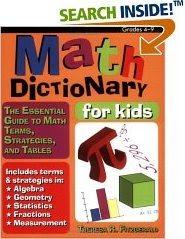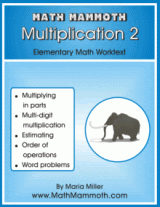## Posts

Showing posts from January, 2008

### Multiplication algorithm poll

Dave at Math Notations has an interesting poll up, till Feb. 29th. It asks about which multiplication algorithm should be taught in grades 3-5 for multidigit multiplication, and if mastery should be required.

If you're interested in that issue, go check it out and vote:

43x27? Which Algorithm should be taught? A MathNotations survey will end the debate!

(The part about ending the debate is mentioned tongue-in-cheek, I'm sure :)

### Should you use a math dictionary?When it comes to what is fashionable in homeschooling, these days lap books, notebooking, lapbooking, whatever term you use, are definitely "in". I haven't seen much anything for math, though. And no, I haven't made any lapbooks either! But I do have an idea for one:

Let your child/student make their own math dictionary! Just make a new page in it every time there is a new concept or term to study. The student can write the term, write an explanation, an example calculation, or draw a picture of it − or all of those.

Making such a math vocabulary book shouldn't take lots of time, because you don't usually encounter new words every single lesson in a typical math curriculum. The rest of the time the book can act as a reference or as a review medium.

There is one exception though, and that is GEOMETRY. In geometry, just about all the time you have new terms to learn. In fact, a big part of the geometry in elementary grades is simply learning the meaning of words …

### New Math Mammoth books: Multiplication 2, Division 2, Place Value 4Some recent additions to Math Mammoth Blue Series books:

Math mammoth Multiplication 2
This book concentrates on multi-digit multiplication, first explaining what it is based on (multiplying in parts), then practicing the algorithm. also included: order of operations, multiplying with money, and lots of word problems.

See sample pages here: (PDF)

Contents & Introduction
Multiply by Whole Tens and Hundreds
Multiply in Parts
Multiplying in Columns, Standard Way
Error of Estimation
Order of Operations
Money and Change
Multiplying 3-digit by 2-digit

Math Mammoth Division 2
This book includes lessons on division, long division, the remainder, part problems, average, and problem solving.

See samples:
Contents and Introduction
Division Terms, Zero and One
Finding Parts with Division
Long Division 1
Long Division with 4-Digit Numbers
Average
Divisibility Rules

NOTE: Multiplication 2 and Division 2 now replace the earlier book called Multiplication Division 2.

Math Mammoth Place Value 4
In this book, the focus is…

### A reminder and games

Henry Cate is still looking for submissions for the Homeschooling Carnival logo contest. If you've been kicking around some ideas, please submit them soon.

Then, a new games website (for me, anyway): Exuberant games!

These are really simple, letting children practice the basic operations (addition and division facts) but in nice child-friendly settings.

Multiplication games are here

For example, my daughter enjoyed serving ice cream to the penguins who would turn sad with wrong answers (the Cone Crazy game).

### Classic math mistakes

I found this link just today, via Mr. Barton's essential math freebies page.

It is a collection of classic math mistakes, presented as posters with silly titles.

You can post them on your wall and let students figure them out, because the poster doesn't actually explain the mistake; it just shows an example of it and the funny title.

Check it out:

Classic Math Mistakes - Gallery of Posters

### Clock face problemDivide the face of the clock into three parts with two lines so that the sum of the numbers in the three parts are equal.
Let's first understand the problem. We need to draw lines into the clock face. It doesn't say the lines need to end in the center or go through the center. The lines could seemingly go many various ways.

Again, to help a student who can't even get started, just tell them to draw some lines into the clock face. Here's one example:

Of course this is not a solution since it does not fulfill the condition that the sum of the numbers in the three parts needs to be equal. But it gets you started. You have some lines, so you can ask the student to add the numbers in the three parts and verify the sums are NOT equal.

How do we make the sums to be equal? Just random trying would take a long time.

The KEY to this problem is that since the sum in each of the three parts is equal, if you add those three sums, you will get the sum of all the numbers in the clock fa…

### Solving the plus sign problem

How many addition signs should be put between digits of the number 987654321 and where should we put them to get a total of 99?
This is a fifth grade problem taken from Word Problems for Kids by Canada's SchoolNet.

The first step, as always, is to understand the problem. The student needs to know what is an "addition sign" and a "digit". We're simply asked to put plus signs in between those numbers and add them up, and try to come up with 99.

Then, after we have a basic idea of what the problem is about, is the time to do something. You know, often the child may say, "I don't know how to start. I have no idea what to do!"

But in this case, as often happens, you'll get somewhere as soon as you'll do something. It's really simple: put some plus signs in there and just see what happens. Let's simply put the plus sign in between every digit:

9 + 8 + 7 + 6 + 5 + 4 + 3 + 2 + 1 = 45.

Great, we got something. We got 45 which is too small…

### Two problems for YOU to solve

I'd like for you to try solve these two 5th grade problems. They are from a very nice collection of word problems for kids by Canada's SchoolNet.

I'd like to use them to illustrate problem solving. But before I do that and give you the solutions, please try to solve them yourself. And don't post solutions here for now; I will have to reject such comments.

Solutions and discussion will follow in a few days.

THE FIRST PROBLEM IS:

* How many addition signs should be put between digits of the number 987654321 and where should we put them to get a total of 99?

THE SECOND IS:

* Divide the face of the clock into three parts with two lines so that the sum of the numbers in the three parts are equal.

### Review of Math Mammoth Geometry 1

Sol Lederman from Wild About Math blog has posted a review of my Math Mammoth Geometry 1 book.

I appreciate the review very much and want to thank Sol for it.

### Teaching problem solving

I have written a more complete presentation on the topic of teaching problem solving — including what not to do. I posted it on my HomeschoolMath.net website:

The do's and dont's of teaching problem solving in math

I'd like your input. For example if you can suggest other resources to be added to the end of that article, or some other ideas, leave a comment here on the blog or email me.

Great news!

The LightBlue Series books are now available as downloads. These are designed to be a complete curriculum for a given grade. They follow the lesson format of my Blue Series books: explanations, then problems (worktext). In fact, the two series do share a lot of material.

The LightBlue is for you if you need a math curriculum for a given grade that covers all the topics needed. These are practically no-prep for the teacher, and in fact, kids can probably teach themselves the majority of the time (assuming they can read).

Math Mammoth Grade 1 Complete Worktext

Math Mammoth Grade 2 Complete Worktext

Math Mammoth Grade 3 Complete Worktext

I will have grade 4 ready sometime this spring, February or March I think.

### Blogging in 2007 and in 2008

Welcome to 2008! Carnival of homeschooling, the 2-year anniversary edition is up at Why Homeschool. Henry notes that during 2007, over 300 bloggers contributed to the carnival. That is quite a bunch! I'm glad, for the hosts' sakes, that not all of them contribute any one time!

Also, they are hosting a contest for the Carnival of Homeschooling graphic.

Also, Sol at Wild About Math has invited math bloggers to find their most popular posts during 2007. Well, I'm kind of a latecomer on this because he wanted the comments before the end of 2007, but I thought maybe you'd enjoy knowing anyway.

Now, I used to have but a measly SiteMeter free version installed, so I frankly don't know which posts were visited the most. (I did install Google Analytics yesterday, instead, so from now on I will know!)

Based on the number of comments, these were among the most popular:

Multiplying in parts and the standard algorithm

So many percent more